# Latest Excel functions which makes your life easier

Updated on Fri Oct 11 2019

SHARE

Microsoft Excel is very popular spreadsheet application software used by a large number people globally.It mainly used for data organizing, manipulation, analyzing and visualizing purposes. There are number of useful Excel functions and they add new functions time to time for make our lives more easier. In this small tutorial I will introduce a few such new formulas which will be very helpful for your day-to-day works.

## IFS ( ) function

This excel function provides a alternative solution for complex nested “IF” function in Excel. We can evaluate multiple conditions and output value pairs more clean and organized way using Microsoft Excel IFS formula. If you have used nested if functions you will be very happy to use Microsoft Excel IFS function instead of nested if functions in Microsoft excel.

Microsoft Excel IFS functions evaluates conditions left to right order and returns the corresponding return value when it finds the first condition which evaluates to true.

The function structure is very simple as you can see in the following figure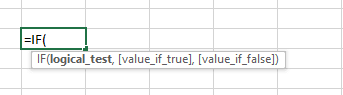IFS function parameters

In the following simple example for assigning the grade based on marks, you can see the application of the Microsoft Excel IFS function.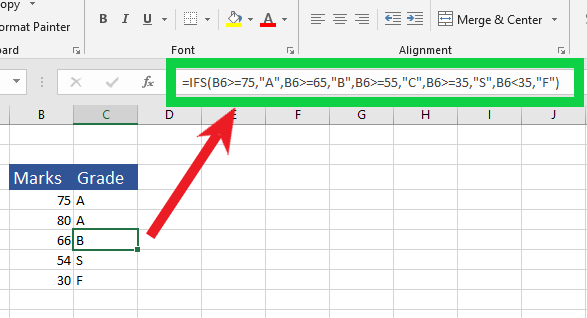Example IFS function application

## SWITCH ( ) function

SWITCH excel function evaluates an expression against a list of values in order, and returns the first matching result. If no results match, the else is returned. This function could also be used instead of nested IF statement.

The function arguments of the Microsoft Excel function are as follows.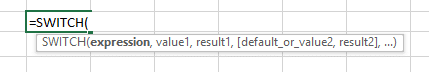SWITCH function parameters

Now let us look at a simple example to learn how to use this Microsoft Excel SWITCH function. Here we check the number in column “B” and then based on that number we get the number in words in the column C.SWITCH function example

## MAXIFS ( ) function

MAXIFS Excel function return the maximum value among cells specified by a given set of conditions or criteria. The criteria or conditions could be in the form of number, text or expression that defines which cells will be evaluated as maximum.

You should be careful to select criteria ranges to be same size and shape as the range that evaluate for maximum value.

This is the general structure of the function.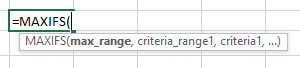MAXIFS function parameters

Now let us look at an example to get a clear idea of how to use this Microsoft Excel MAXIFS function.MAXIFS function example

Criteria ranges does not need to be adjacent columns or same rows as max_range, only thing is, it must be same size and shape. In this example I have used only text criteria,but you are free to use numbers or valid expressions in your application.

## MINIFS () function

MINIFS Excel function returns the minimum or smaller value among cells specified by a given set of conditions or criteria. It is very similar to the above discussed MAXIFS function.

Function structure is as follows;MINIFS function parameters

In the following example we are going to get the lowest of the marks obtained by female student who got “A” grades.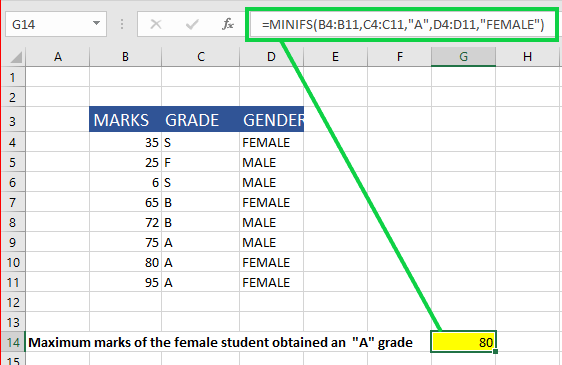MINIFS function example

## CONCAT () function

CONCAT Excel function is mostly similar to Microsoft Excels CONCATENATE function, only being better, shorter and easier. It joins a list or range of text strings into one text string. Older CONCATENATE function only accepts cell references as its inputs in addition to texts. But CONCATENATE function accepts range references also.

It has very simple function structure which accepts texts,cell references or range reference as its parameters.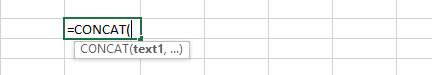CONCAT function parameters

Excel CONCAT function will help you to manipulate your text based data like joining parts of addresses, contact numbers or names. Following simple example could be used as a guide for application of CONCAT function in real situations.Excel CONCAT function example

## TEXTJOIN () function

TEXTJOIN Excel function is somewhat similar to CONCAT function. The difference is TEXTJOIN function allows you to define a text delimiter and then the function automatically add that specified delimiter in between each text that you intended to join.

In addition, the TEXTJOIN function allows you to decide whether to ignore or not the empty cells found in the range or list of texts.

Now let us look at the function structure.TEXTJOIN function paramters

delimiter : It can be any of the valid character, which you have to enter within quotation mark.

ignore_empty : This parameter should be set to “TRUE” or “FALSE” based on whether or not to ignore the empty values found in your list or range of values.

Then you can input raw text values entered inside quotation marks separated by commas or input cell references separated by commas or range reference to your list of texts.

Look at the following example for further understand the application of Microsoft Excel TEXTJOIN function.TEXTJOIN function example